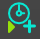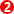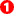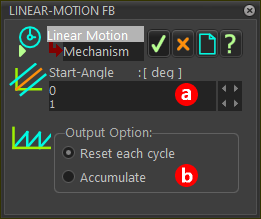﻿ MechDesigner Reference & User Interface > Dialog-Boxes > Dialog: Function-Block: Linear-Motion

# Dialog: Function-Block: Linear-Motion

Navigation:  MechDesigner Reference & User Interface > Dialog-Boxes >

# Dialog: Function-Block: Linear-Motion

## Linear-Motion FB

The default motion-values at the output from the Linear-Motion FB are equal to the Master-Machine-Angle (MMA).

Use the output from the Linear-Motion FB as the machine-angle at the input to other Kinematic FBs.

Use the Linear-Motion dialog-box to edit the output relative to the Master-Machine-Angle.

### Open Linear-Motion dialog-boxEdit the Linear-Motion FB:

 1.Double-click the Linear-Motion FB in the graphic-area. - or - 1.

The Linear-Motion FB dialog-box is now open.

### Linear-Motion dialog-box

 The function of the Linear-Motion FB is: If Reset: Output  = MMA + start-angleor If Accumulate: Output = (Revs × 360) + Master Machine Angle + Start-AngleLinear Motion FB dialog-box

Start-Angle:Enter a real number in the data-boxA positive value will advance the timing relative to the MMA.

Output Options:◉ Reset each Cycle (default) Output = Master Machine Angle +◉ Accumulate Output = (Revs × 360) + Master Machine Angle +